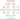# Merge Sort Algorithm

May 17, 2019This algorithm is very efficient one, and is classic example of Divide and Conquer algorithms.

In this algorithn, we divide the whole array into smaller sub-problems. i.e. till we get only single element. Single element means, there is no need to sort it. Lets call it sorted sub-array.

Next step is to merge two such sorted sub-array. The operation is called merge two sorted arrays.

As, the recursion stacks pops-up. We keeps on merging sorted sub-arrays. Untill all sub-arrays merged into one.

Finally we get the sorted array.

## Merge Sort Algorithm

• First method takes array and index of array as first and last index.
• Divide the array into two equal half.
• Recursive call to same function with the left half array.
• Recursive call to same function with the right half array.
• Now, we get sorted sub-array. Note: each single element is itself sorted.
• Next step is to merge two sorted sub-arrays.
• We keep on doing this, untill all sub-arrays merged to become one.

See the code here:

``````public void doMergeSort(int[] arr, int l, int r) {
if (l < r) {
int m = (r+l)/2;

doMergeSort(arr, l, m);
doMergeSort(arr, m+1, r);

merge(arr, l, m, r);
}
}

private void merge(int[] a, int l, int m, int r) {
int[] left = new int[m-l+1];
int[] right = new int[r-m];

for (int i=l; i<=m; i++) {
left[i-l] = a[i];
}
for (int i=m+1; i<=r; i++) {
right[i-m-1] = a[i];
}

int lindex = 0;
int rindex = 0;
for (int i=l; i<=r; i++) {
if (lindex < left.length && (rindex >= right.length || left[lindex] < right[rindex])) {
a[i] = left[lindex];
lindex ++;
}
else {
a[i] = right[rindex];
rindex ++;
}
}
}``````

In above merge method, I have handled all cases in same loop. There is an alternate solution to this as well, see below:

``````public void merge2(int[] a, int l, int m, int r) {
int l1 = m-l+1;
int l2 = r-m;
int[] left = new int[l1];
int[] right = new int[l2];

for (int i=0; i<l1; i++) {
left[i] = a[l+i];
}
for (int i=0; i<l2; i++) {
right[i] = a[m+1+i];
}

int lindex = 0;
int rindex = 0;
int i=l;
while (lindex < l1 && rindex < l2) {
if (left[lindex] < right[rindex]) {
a[i] = left[lindex];
lindex++;
}
else {
a[i] = right[rindex];
rindex++;
}
i++;
}

//copy the leftover array
for (int j=lindex; j<l1; j++) {
a[i] = left[j];
i++;
}
for (int j=rindex; j<l2; j++) {
a[i] = right[j];
j++;
}
}``````

## Graphical Example## Key Points

• Its a great algorithm to sort Link lists, because this algorithm does not rely on accessing values by indexes.
• It takes extra memory in merge method.
• Very efficient in sorting large number set
• Performance is very good. its complexity is O(n log n)
• Based on Divice and Conquer technique
• Its a stable sort algorithm

## Runtime complexity

The algorithm runs on O(n log n) in worst/average case.

The merge function runs in O(n) time. Lets look at doMergeSort method which divides the input array. It divides the array into equal halves each time. First n/2, then (n/2)/2=n/4, then n/8, then n/16 and so on.

Which evaluates to log n So, total complexity of O(n log n)